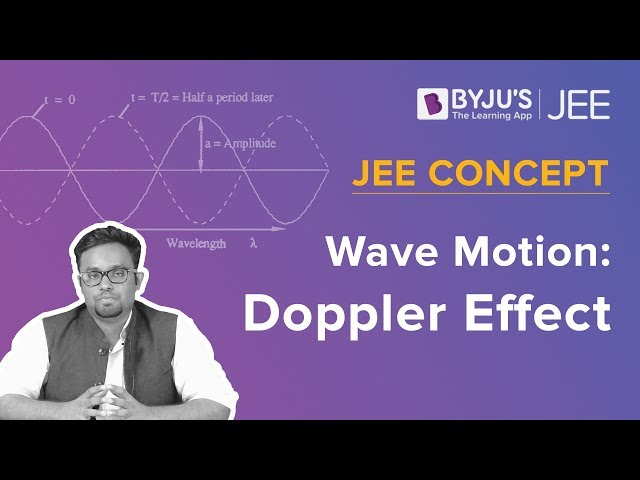# Doppler Shift

Wave motion is a key concept in Physics that can be understood in various ways. The change in frequency of a wave in relation to an observer who is moving relative to the wave source is explained by the Doppler shift or Doppler effect. In this article, let us learn in detail about the Doppler shift.

## What is Doppler Shift?

The Doppler shift, also known as the Doppler effect, is defined as the change in the wavelength or frequency of the waves with respect to the observer who is in motion relative to the wave source. The Doppler shift was theorised in the year 1842 by the Austrian physicist Christian Doppler.

## What is the Doppler Shift Formula

 $$\begin{array}{l}f=(\frac{c\pm v_{r}}{c\pm v_{s}})f_{0}\end{array}$$

Where,

• c is the velocity of waves in the medium
• vis the velocity of the receiver relative to the medium (positive if the receiver is moving towards the source and negative if in the opposite direction)
• vis the velocity of the source relative to the medium (positive if the source is moving away from the receiver and negative if in the opposite direction)
• f is the observed frequency
• fis the emitted frequency

Above is the Doppler shift or Doppler effect formula explaining the relationship between observed frequency and the emitted frequency where the speed of source and receiver is lower than the velocity of the waves in the medium.

Following is the formula when the speeds of receiver and source are comparatively lower than the speed of the wave:

Observed frequency:

 $$\begin{array}{l}f=(1+\frac{\Delta v}{c})f_{0}\end{array}$$

Change in frequency:

 $$\begin{array}{l}\Delta f=\frac{\Delta v}{c}f_{0}\end{array}$$

Where,

$$\begin{array}{l}\Delta f=f-f_{0}\end{array}$$
$$\begin{array}{l}\Delta v=v_{r}-v_{s}\end{array}$$
velocity of the receiver relative to the source (positive when the source and the receiver moving towards each other)

Related Articles:

To know more about the doppler effect, click on the video below.## Application of Doppler effect

#### Sirens

The principle behind the siren is that it starts at a higher pitch than its stationary pitch when it slides down from the observer, and again when it recedes from the observer, it continues at a lower pitch than its stationary pitch. It is used in emergency vehicles. Siren’s velocity is given as

$$\begin{array}{l}v_{radial}=v_{s}.cos\Theta\end{array}$$
where Ө is the angle between the object’s line of sight and the forward velocity.

#### Astronomy

In astronomy, the Doppler effect for electromagnetic waves of light results in either a red-shift or a blue-shift. With the help of the Doppler effect and radial velocity, one can measure the speed at which stars or galaxies are receding or approaching us.

#### Velocity Profile Measurement

Ultrasonic Doppler Velocimetry is used to measure the real-time completion velocity profile of any liquid containing suspended particles like dust, emulsions and gas bubbles. The flow can be pulsating, laminar or turbulent, oscillating or stationary.

## Frequently Asked Questions – FAQs

Q1

### What is a Doppler Shift?

The Doppler Shift or the Doppler Effect is the change in frequency of a wave in relation to an observer who is moving relative to the wave source.
Q2

### What is the difference between the Doppler shift and the Doppler Effect?

More precisely, the term Doppler effect refers to the change in the observed frequency of a wave when the source and the observer move relative to the medium. Doppler shift is the movement of the source or observer with respect to the medium.
Q3

### What causes the Doppler Effect?

The Doppler effect is described as the effect produced by a moving source of waves in which there is an apparent upward shift in frequency for observers towards whom the source is approaching and an apparent downward shift in frequency for observers from whom the source is receding.
Q4

### What does a large Doppler shift indicate?

A large Doppler shift indicates the moving object generating light or sound waves is moving very fast.
Q5

### Why is the Doppler shift important for astronomy?

In astronomy, the Doppler effect for electromagnetic waves of light results in either a red-shift or a blue-shift. With the help of the Doppler effect and radial velocity, one can measure the speed at which stars or galaxies are receding or approaching us.
Stay tuned with BYJU’S for more such interesting articles. Also, register to “BYJU’S – The Learning App” for loads of interactive, engaging Physics-related videos and unlimited academic assistance.

Test your knowledge on Doppler shift